# 程序执行的本质

CPU分为寄存器，运算器和控制器三部分。

# 第一行c++代码

``````#include <iostream>

int main()
{
std::cout << "Hello World!\n";
}``````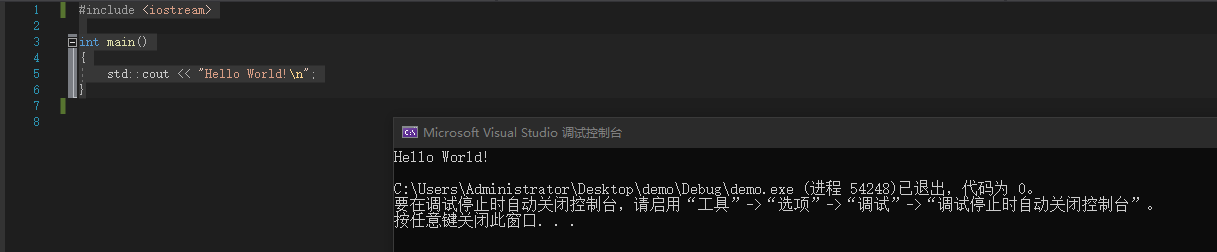# 常用语法

c++的源文件扩展名是cpp

c++程序的入口是main函数

c++完全兼容c

## cin、cout

cout表示输出，例如：

``````#include <iostream>
using namespace std;

int main()
{
cout << "Hello World!\n";
return 0;
}
``````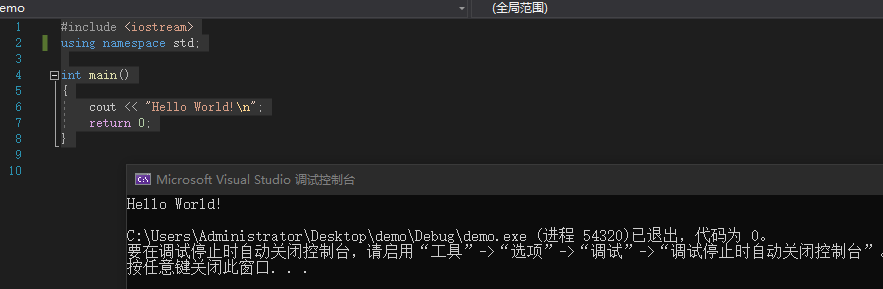``````#include <iostream>
using namespace std;

int main()
{
cout << "Hello World!" << endl;
return 0;
}``````

cin表示从键盘输入，例如：

``````#include <iostream>
using namespace std;

int main()
{
int age;
cin >> age;
cout << "age is: " << age << endl;
return 0;
}
``````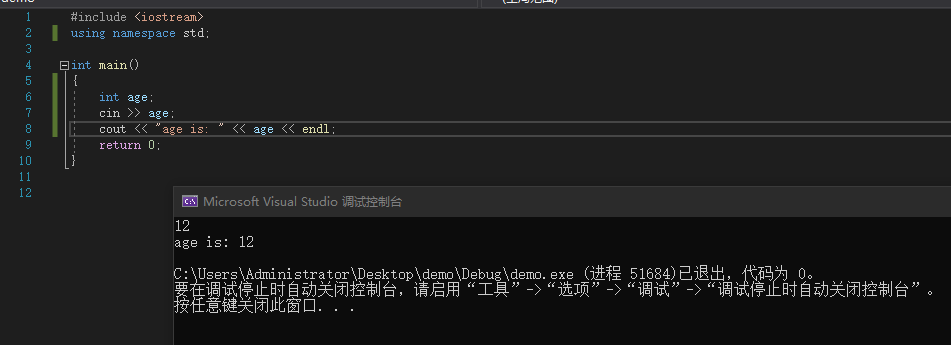## getchar()

``````#include <iostream>
using namespace std;

int sum(int v1, int v2) {
return v1 + v2;
}

int sum(int v1, int v2, int v3) {
return v1 + v2 + v3;
}

int main()
{
cout << sum(10, 20) << endl;
cout << sum(10, 20, 30) << endl;
return 0;
}
``````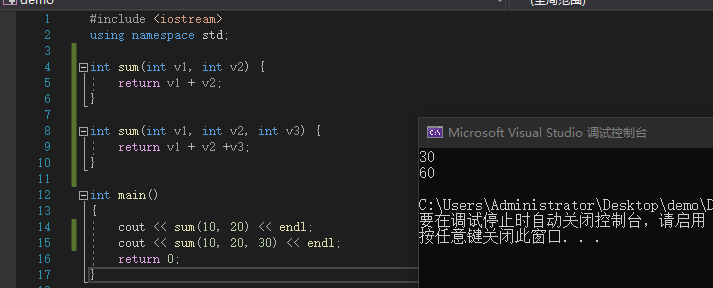sum函数随着传入的实参个数不同会自动寻找对应的函数去计算。

# 默认参数

c++允许函数设置默认参数，在调用时可以根据情况省略实参，规则如下：

• 默认参数只能按照右到左的顺序
• 如果函数同时有声明，实现，默认参数只能放在函数声明中
• 默认参数的值可以时常量，全局符号（全局变量，函数名）

``````#include <iostream>
using namespace std;

int sum(int v1 = 5, int v2 = 6) {
return v1 + v2;
}

int main()
{
cout << sum() << endl;
cout << sum(10) << endl;
cout << sum(10, 20) << endl;
return 0;
}
``````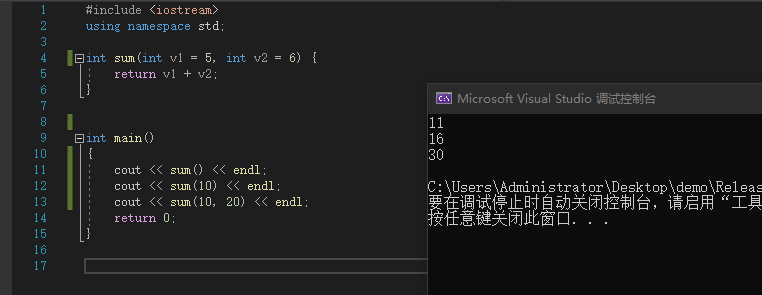# extern “C”

``````#include <iostream>
using namespace std;

extern "C" {
void func() {

}

void func(int v1) {

}
}

int main()
{

return 0;
}``````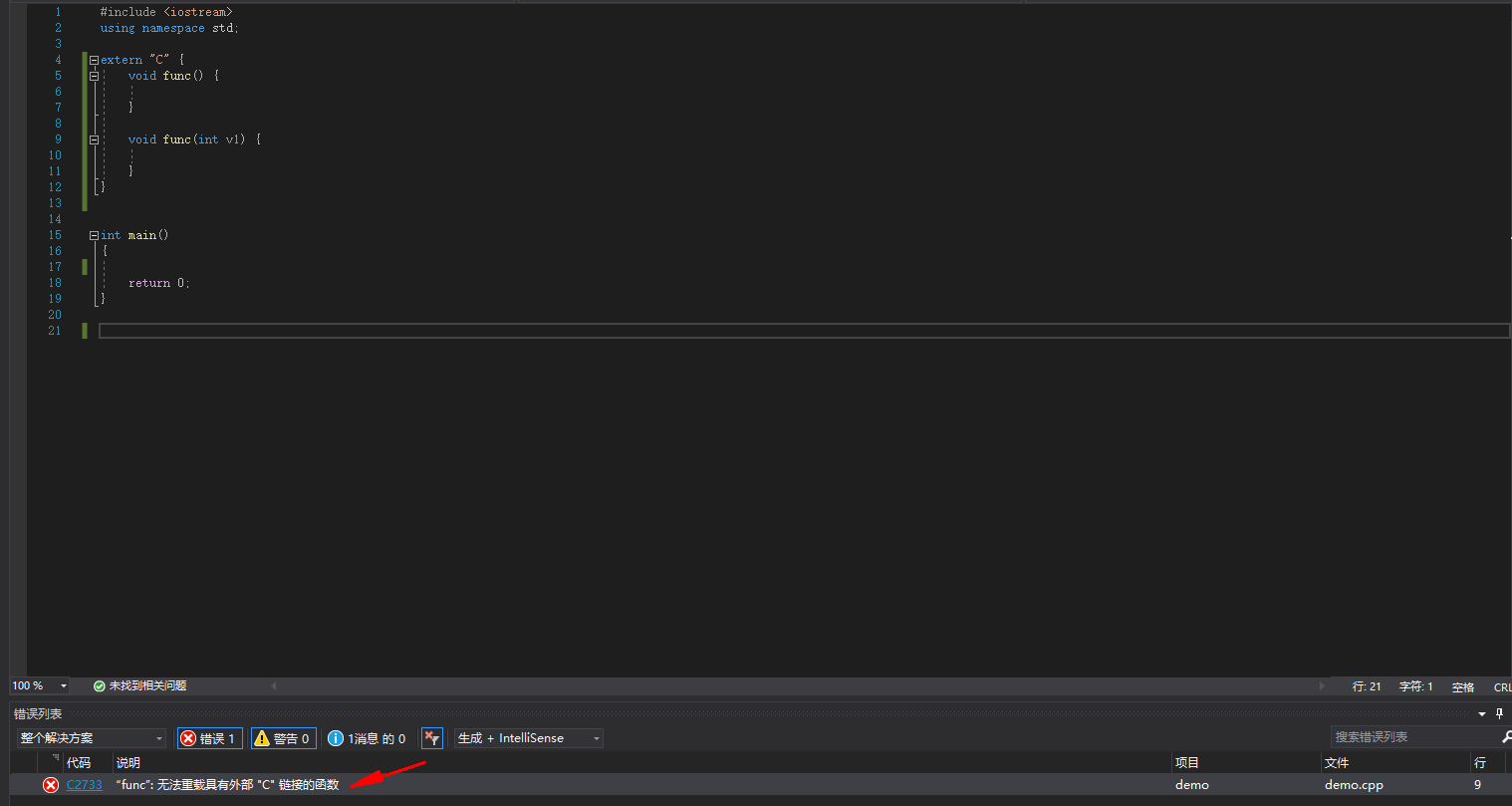• 如果函数通过是有声明和实现，要让函数声明被`extern "c"`修饰，函数实现可以不修饰。

1. 用在c，c++的混合开发中，如果用到一些第三方的c语言库，那么就可以使用 extern c

``````#include <math.h>

int sum(int v1, int v2) {
return v1 + v2;
}

int delta(int v1, int v2) {
return v1 - v2;
}``````

``````#include <iostream>
using namespace std;

extern "C"{
int sum(int v1, int v2);
int delta(int v1, int v2);
}

int main(){
cout << sum(10, 20) << endl;
cout << delta(50, 10) << endl;
return 0;
}
``````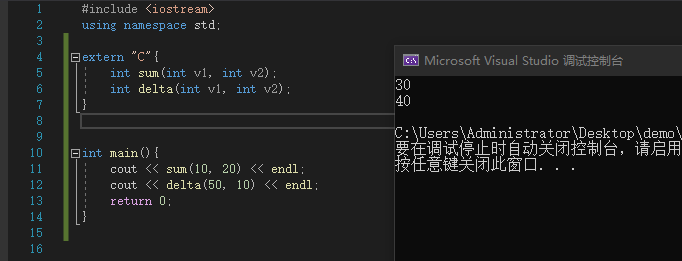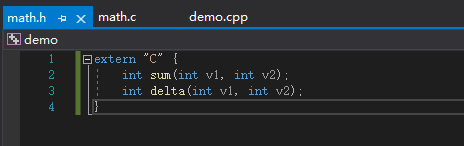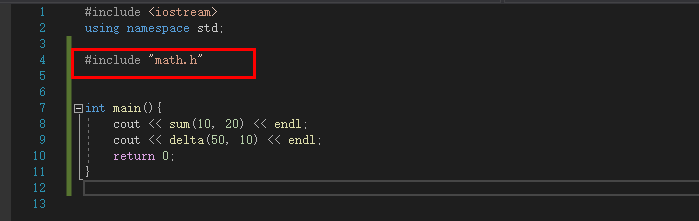# ifdef

c++文件默认都会有一个宏定义 #define __cplusplus

``````#ifdef __cplusplus
extern "C" {
#endif

int sum(int v1, int v2);
int delta(int v1, int v2);

#ifdef __cplusplus
}
#endif``````

# pragma once

`# pragma once`是用来防止头文件被重复包含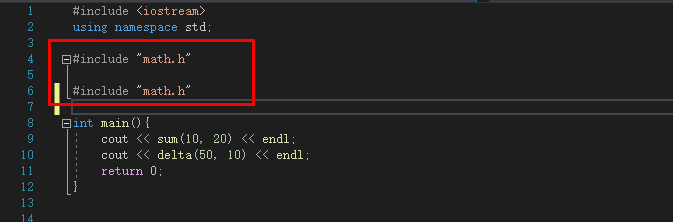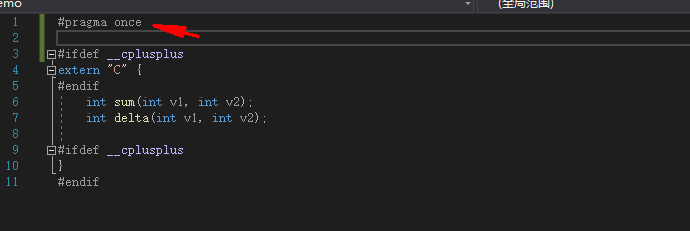# 内联函数

• 编译器会将函数调用直接展开为函数体代码
• 可以减少函数调用的开销
• 会增大代码体积

• 尽量不要内联超过10行代码的函数
• 有些函数即使声明为`inline`,也不一定会被编译器内联，比如递归函数

• 内联函数和宏，都可以减少函数调用的开销

``````#include <iostream>
using namespace std;

#define sum(v1,v2) v1 + v2

int main(){
int c = sum(10, 20);
cout << c << endl;
return 0;
}``````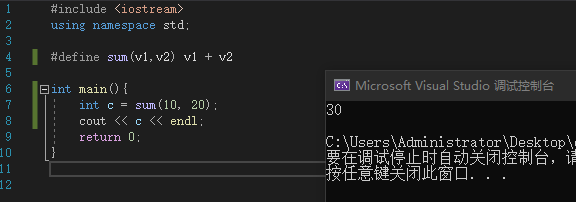• 对比宏，内联函数多了语法检测和函数特性
• 宏只是简单的文本替换，所以遇到计算尽量使用函数

# const

const是常量的意思，被其修饰的变量不可修改。

• 如果修饰的是类、结构体（的指针），其成员也不可以更改
• const修饰的其右边的内容

``````int age = 10;
const int *p0 = &age;
int const *p1 = &age;
int * const p2 = &age;``````

``````#include <iostream>
using namespace std;

int main(){

int age = 10;
int heigh = 20;

int * const p2 = &age;

*p2 = 30; //age=30
p2 = &heigh;  //报错
*p2 = 40; //heigh = 40

return 0;
}
``````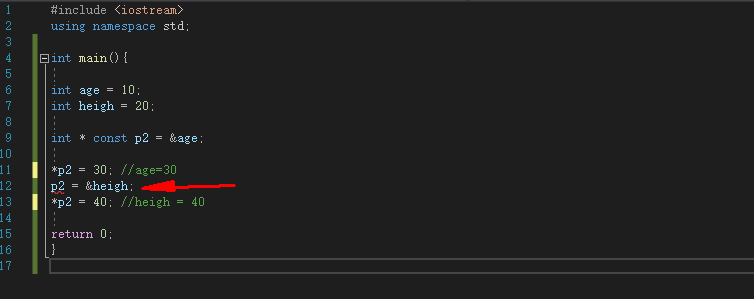# 引用(reference)

``````#include <iostream>
using namespace std;

int main(){

int age = 10;

int* p = &age;

*p = 20;

cout << age << endl;

return 0;
}
``````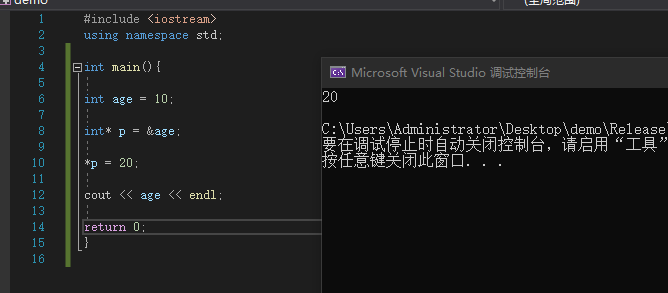``````#include <iostream>
using namespace std;

int main(){

int age = 10;

//定义一个age的引用
int &refAge = age;
refAge = 20;
cout << age << endl;

return 0;
}``````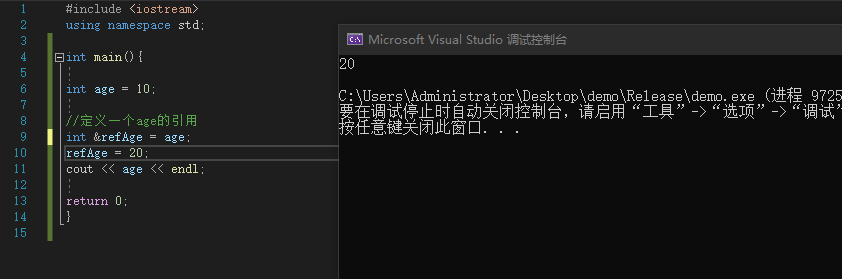• 引用相当于是变量的别名（基本数据类型、枚举、结构体、类、指针、数组等，都可以有引用）
• 对引用做计算，就是对引用所指向的变量做计算
• 在定义的时候就必须初始化，一旦指向了某个变量，就不可以再改变，“从一而终”
• 可以利用引用初始化另一个引用，相当于某个变量的多个别名
• 不存在【引用的引用、指向引用的指针、引用数组】

``````#include <iostream>
using namespace std;

void swap(int &v1, int &v2) {
int tmp = v1;
v1 = v2;
v2 = tmp;
}

int main(){
int a = 10;
int b = 20;
swap(a, b);
cout << "a= " << a << "  b= " << b << endl;
return 0;
}``````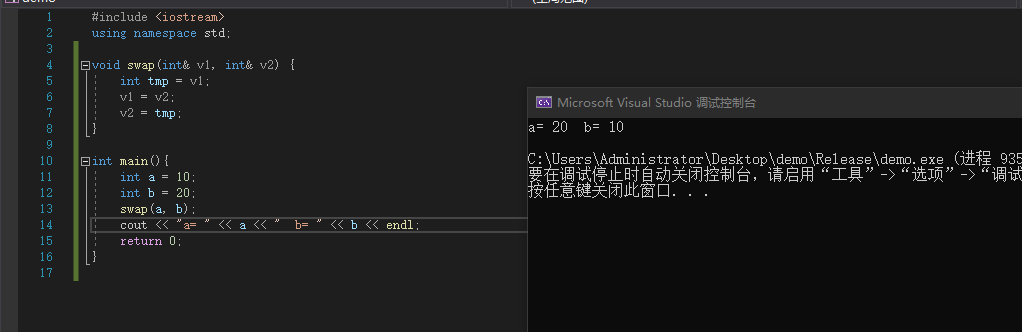# 汇编

• 8086汇编（16bit）
• x86汇编（32bit）
• x64汇编（64bit）
• ARM汇编（嵌入式、移动设备

## 寄存器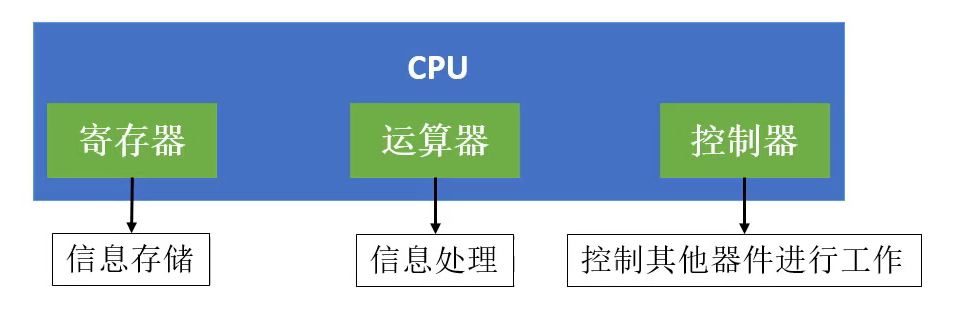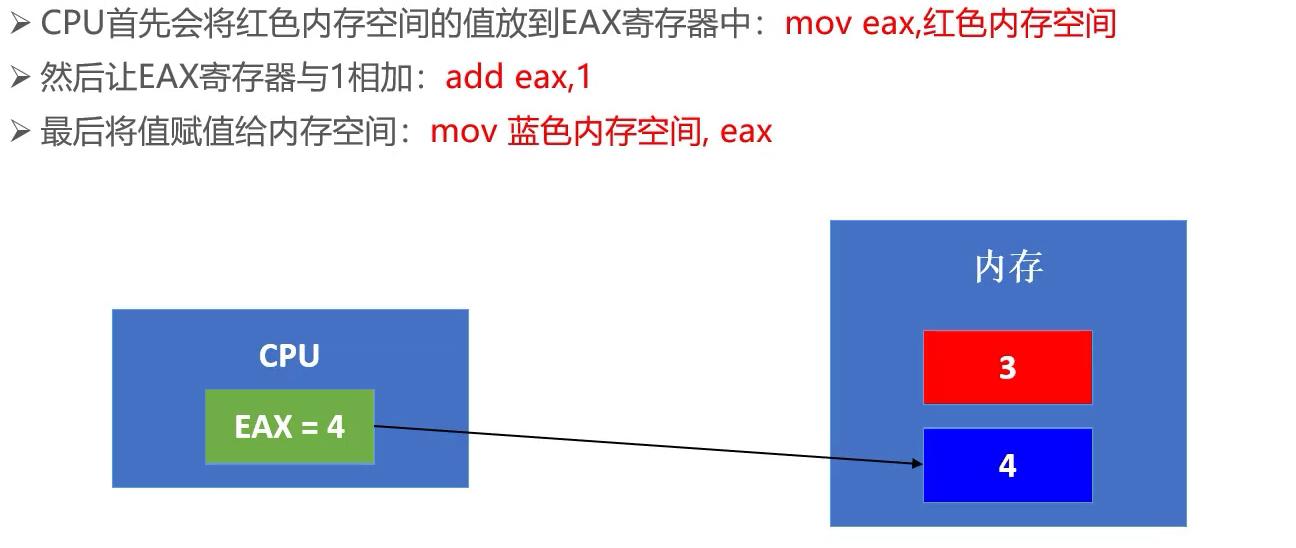## x64汇编-寄存器

RAX RBX RCX RDX 为通用寄存器

X64架构的寄存器，一个寄存器可以存8个字节的数据

x86架构下的通用寄存器为：EAX EBX ECX EDX ,为了兼容32位的CPU架构，拿出了最低四个字节来存放EAX的数据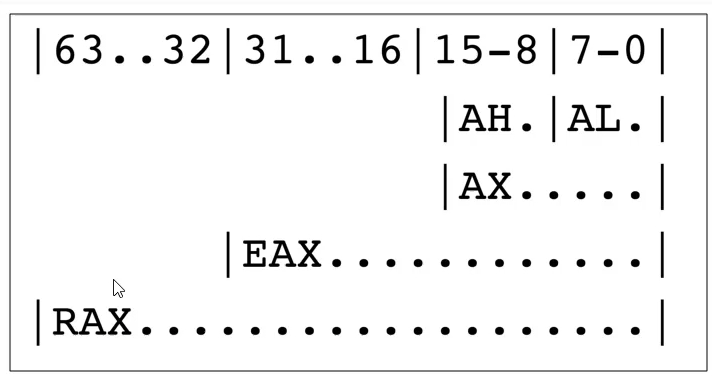## 内联汇编

``````#include <iostream>
using namespace std;

int main(){
int a = 10;

__asm {
mov eax, 10

}

return 0;
}``````

## mov指令

`mov dest, src`

`[ 地址值 ]`

``mov dword ptr [a],0Ah  ``

## call函数地址

call 表示调用函数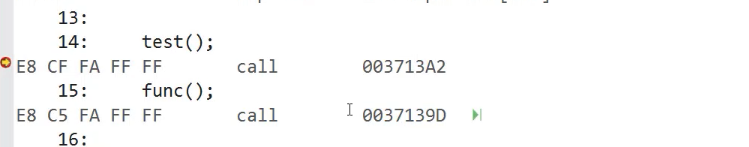## lea指令

`lea dest, [ 地址值 ]`

lea是直接赋值地址值，而mov是取内存地址中存放的东西

``````mov eax, dword ptr [1122H]

lea eax, [1122H]

ret表示函数返回

## xor

`xor op1, op2`

`add op1, op2`

## sub

`sub op1, op2`

## inc

` inc op`

`dec op`

## jmp

jmp 内存地址

j开头的一般都是跳转，大多数是带条件的跳转，一般跟test、cmp等指令配合使用

# 面向对象

## 类的定义

C++中可以使用struct、class来定义一个类
struct和class的区别:
struct的默认成员权限是public
class的默认成员权限是private

``````#include <iostream>
using namespace std;

//类的定义
struct Person{
//成员变量
int m_age;
//成员函数
void run() {
cout << m_age << "run" << endl;
}
};``````

``````class Person {
public:
//成员变量
int m_age;
//成员函数
void run() {
cout << m_age << "run" << endl;
}
};``````

## 类的实例化

``````int main(){
Person person;
person.m_age = 20;
person.run();
return 0;
}``````

## 对象的内存布局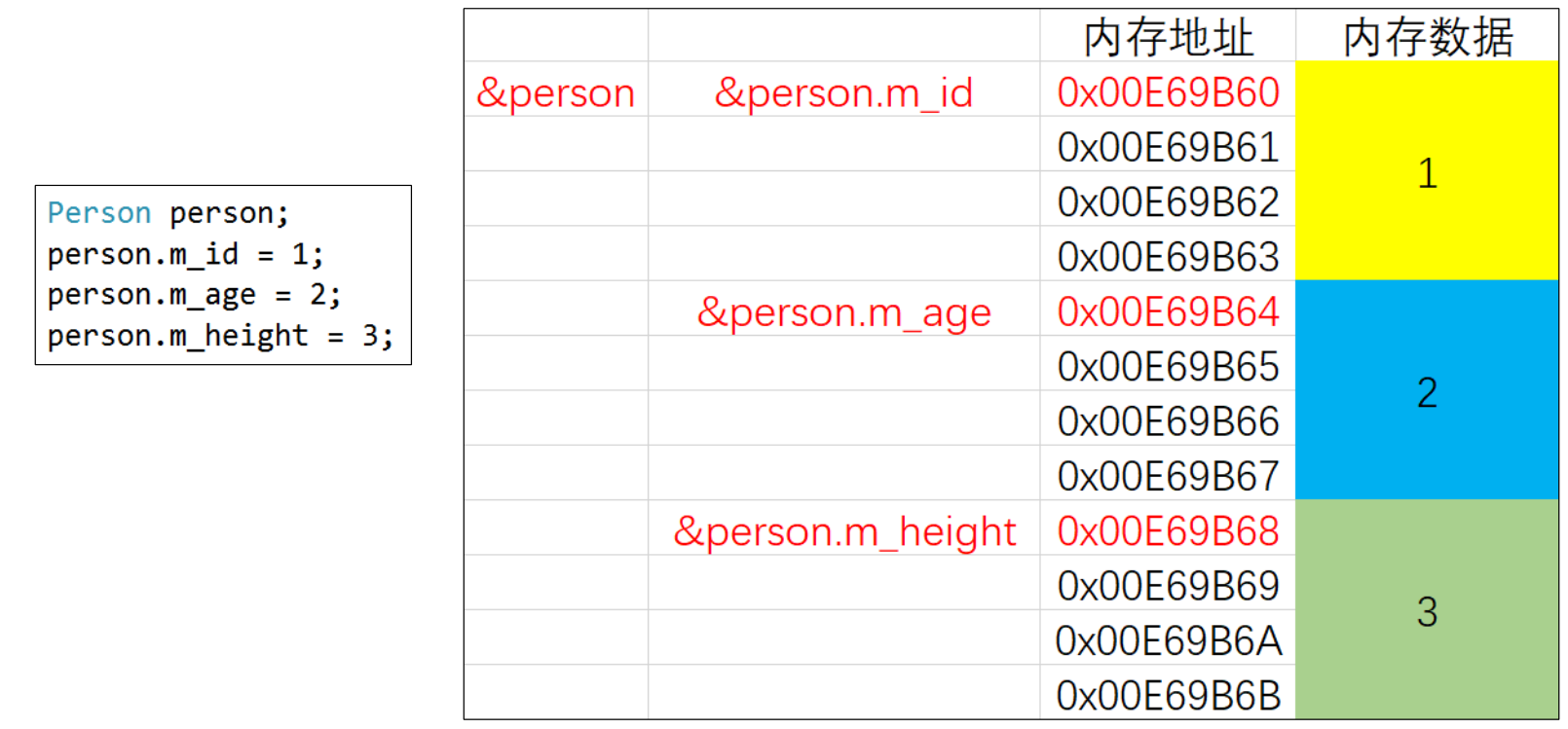## this

this是指向当前对象的指针

``````#include <iostream>
using namespace std;

//类的定义
class Person {
public:
//成员变量
int m_age;
//成员函数
void run() {
cout << this->m_age << "run()" << endl;
}
};
int main(){
Person person1;
person1.m_age = 20;
person1.run();

Person person2;
person2.m_age = 30;
person2.run();
return 0;
}``````

``````#include <iostream>
using namespace std;

//类的定义
class Person {
public:
//成员变量
int m_age;
//成员函数
void run() {
cout << m_age << "run()" << endl;
}
};
int main(){
Person person1;
person1.m_age = 20;
person1.run();

Person person2;
person2.m_age = 30;
person2.run();
return 0;
}``````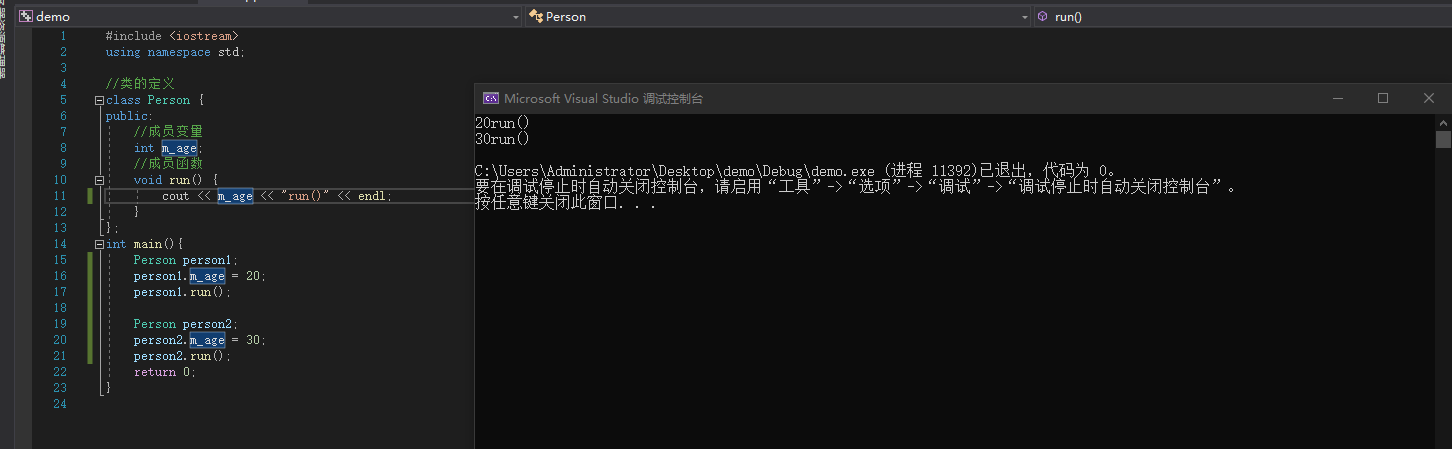## 封装

``````#include <iostream>
using namespace std;

//类的定义
class Person {
private:
//成员变量
int m_age;
public:
void setAge(int age) {
if (age<=0){
m_age = 1;
}
else {
m_age = age;
}
}
int getAge(){
return m_age;
}
};
int main(){
Person person;
person.setAge(-4);
cout << person.getAge() << endl;

return 0;
}``````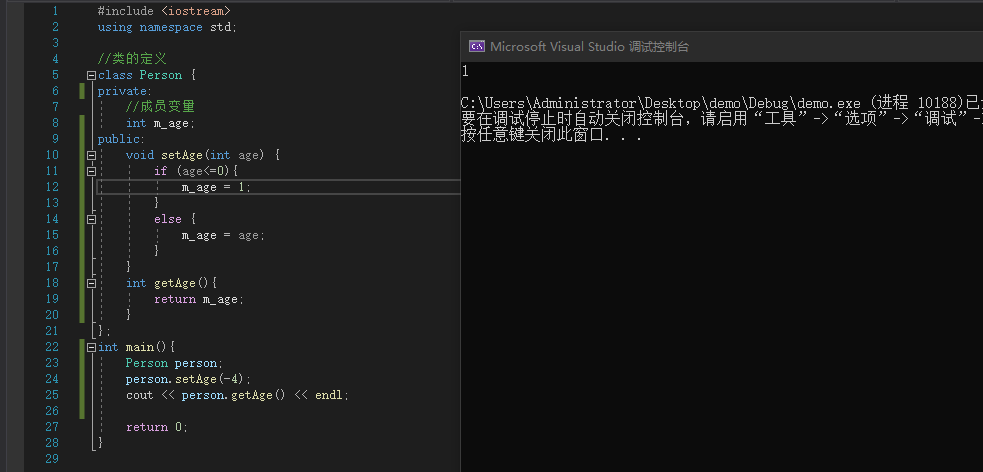## 内存空间布局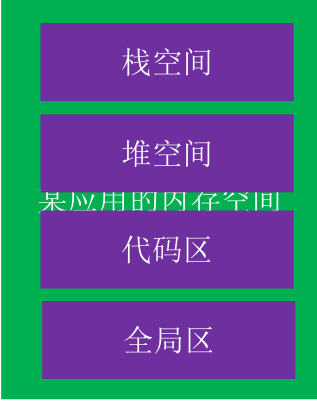## 堆空间

``````#include <iostream>
using namespace std;

int main(){
//申请四个字节的内存空间用来存储整形数据，
//由于malloc申请的内存默认都为void * ，所以需要强转
int * p = (int *) malloc(4);
* p = 11;
cout << * p << endl;
free(p);
return 0;
}
``````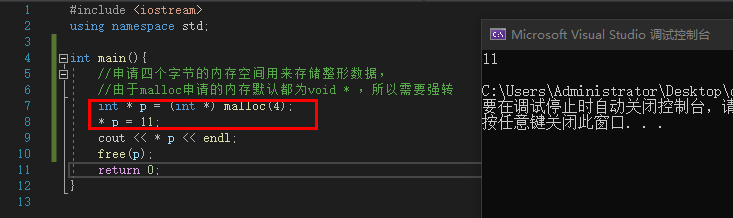``````#include <iostream>
using namespace std;

int main(){

char * p = (char *) malloc(4);
*p = 'a';
*(p + 1) = 10;
*(p + 2) = 11;
*(p + 3) = 12;
cout << * p << endl;
free(p);
return 0;
}
``````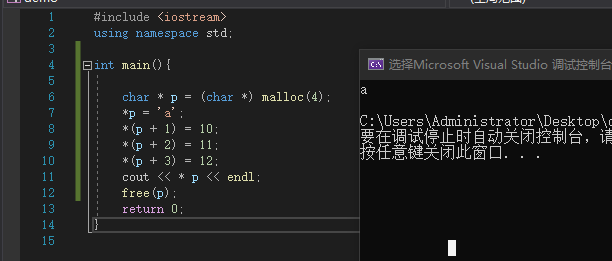``````#include <iostream>
using namespace std;

int main(){

int *p = new int;
*p = 10;
cout << * p << endl;
delete p;
return 0;
}
``````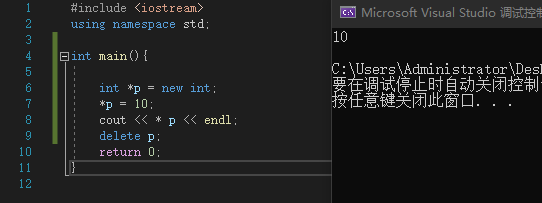• 申请堆空间成功后，会返回那一段内存空间的地址
• 申请和释放必须是1对1的关系，不然可能会存在内存泄露

## 堆空间的初始化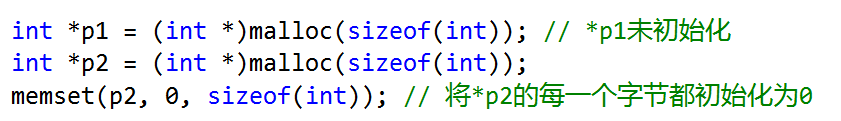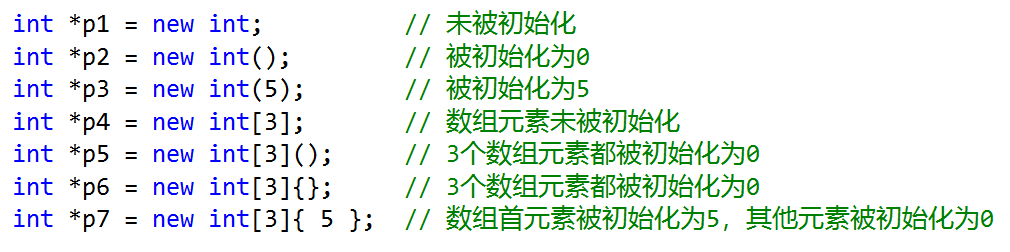memset函数是将较大的数据结构（比如对象、数组等）内存清零的比较快的方法。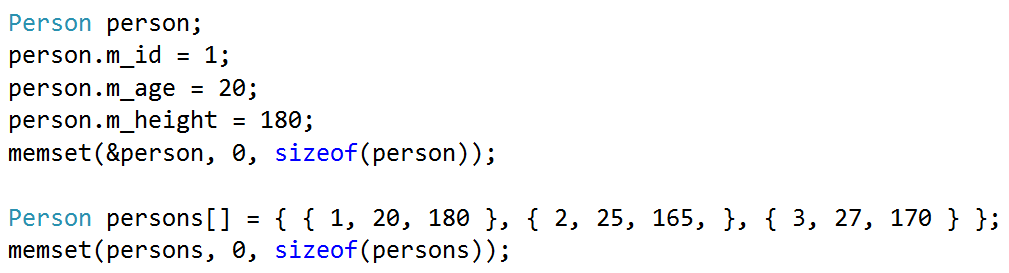## 对象的内存

• 全局区（数据段）：全局变量
• 栈空间：函数里面的局部变量
• 堆空间：动态申请内存（malloc、new等）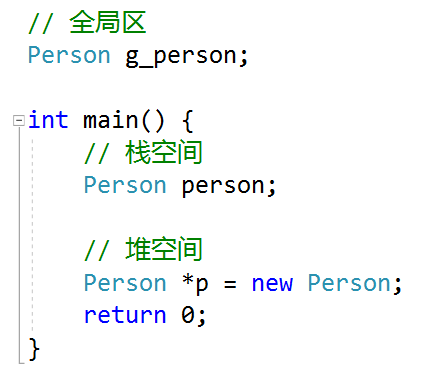## 构造函数（Constructor）

``````#include <iostream>
using namespace std;

class Person {
public:
int m_age;
Person() {
cout << "Person()" << endl;
}
};

int main(){
Person person;
Person person1;
Person person2;
return 0;
}``````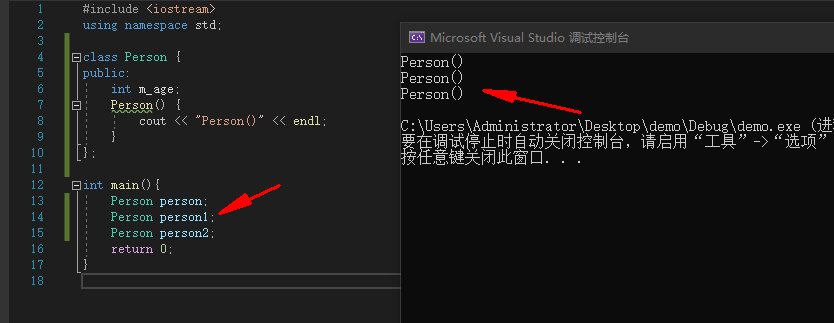• 函数名与类同名，无返回值（void都不能写），可以有参数，可以重载，可以有多个构造函数
• 一旦自定义了构造函数，必须用其中一个自定义的构造函数来初始化对象

## 析构函数（Destructor）

``````#include <iostream>
using namespace std;

class Person {
public:
int m_age;
Person() {
cout << "Person()" << endl;
}

//一个Person对象销毁的象征
~Person() {
cout << "~Persoon()" << endl;
}
};

int main(){
Person person;
Person person1;
Person person2;
return 0;
}
``````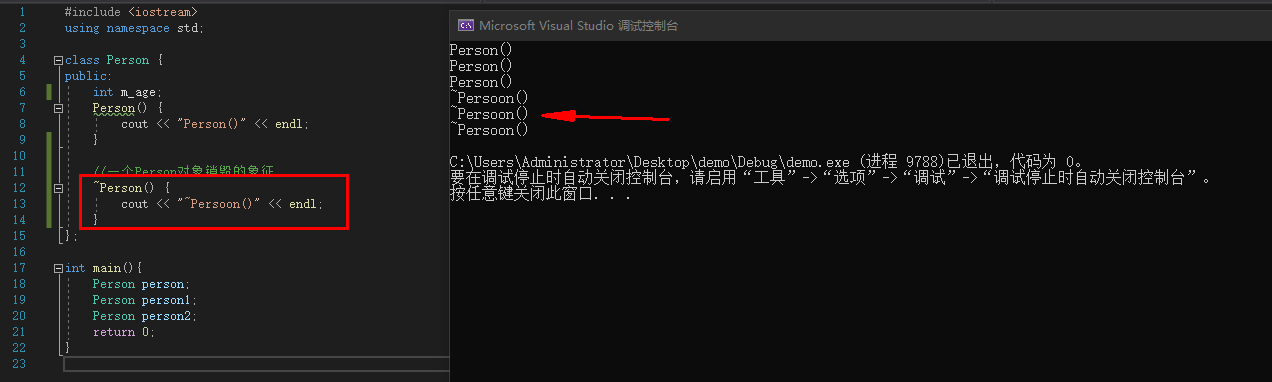• 通过malloc分配的对象free的时候不会调用析构函数
• 构造函数、析构函数要声明为public，才能被外界正常使用

## 声明和实现分离

``````#include <iostream>
using namespace std;

//声明
class Person {
private:
int m_age;
public:
void setAge(int age);
int getAge();
Person();
~Person();
};

//实现
Person::Person() {
m_age = 0;
}

void Person::setAge(int age) {
this->m_age = age;
}

int Person::getAge() {
return this->m_age;
}

Person::~Person() {

}

int main() {
Person person;
person.setAge(1);
cout << person.getAge() << endl;
}``````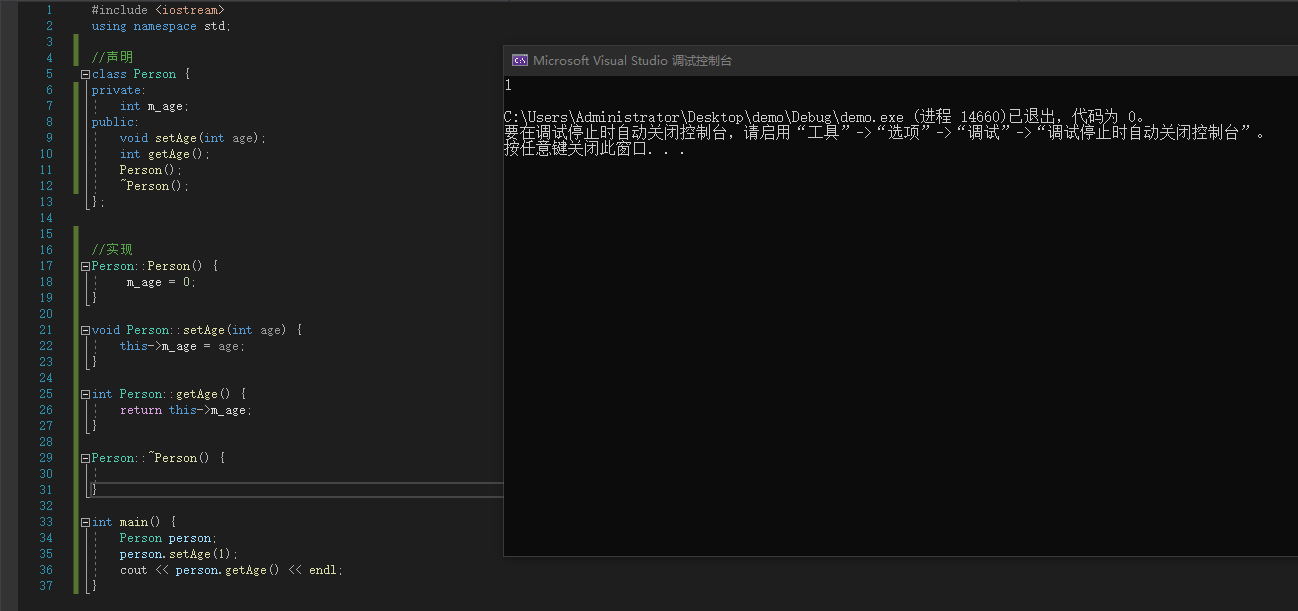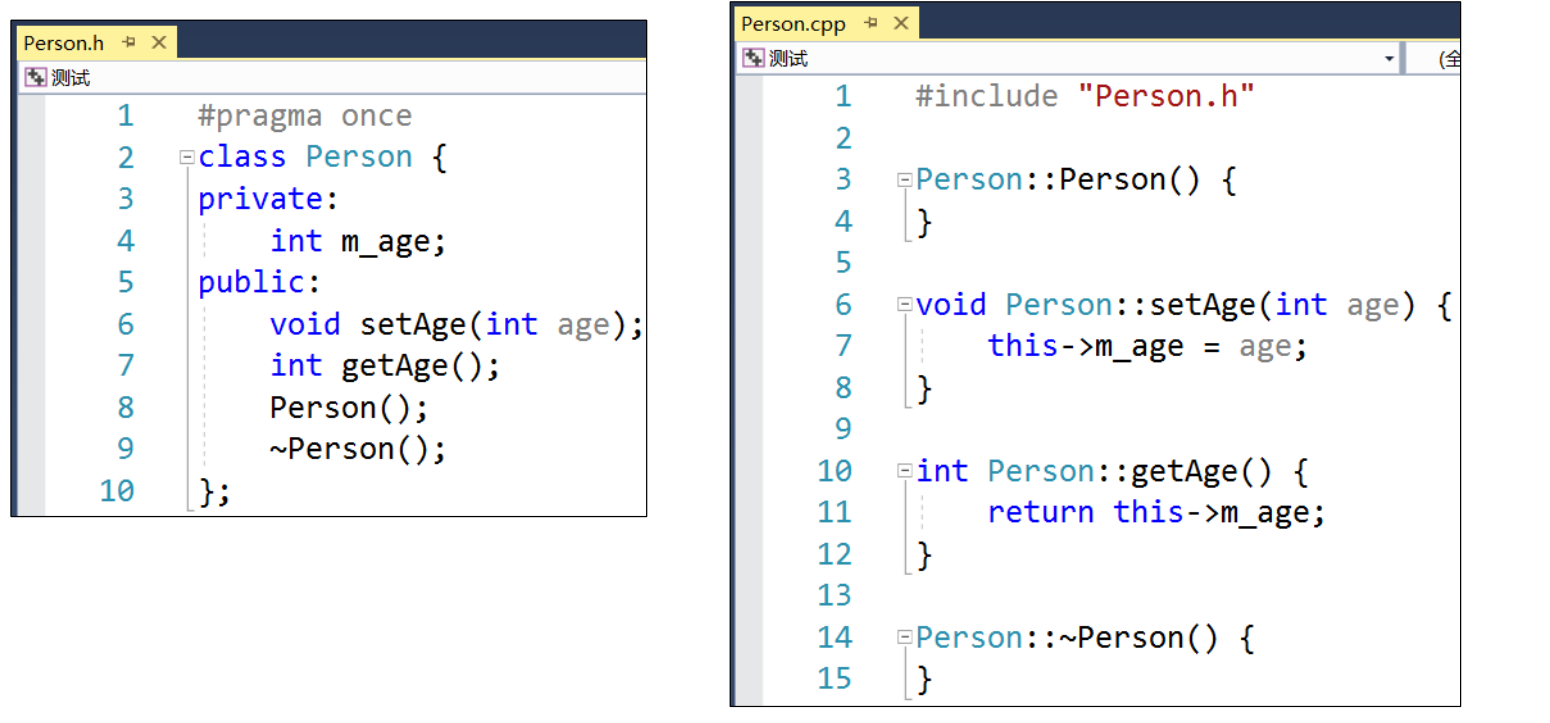## 命名空间

``````#include <iostream>
using namespace std;

namespace abc {
int g_age;

class Person {};

void test() {

}
}

int main() {
abc::g_age = 20;
abc::Person* p = new abc::Person();
abc::test();
return 0;
}``````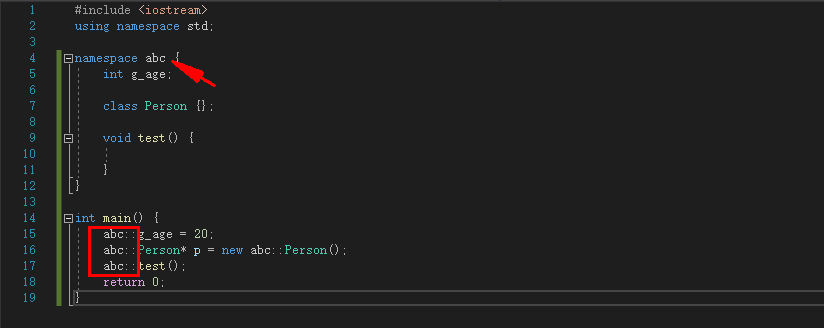``````using namespace abc;
g_age = 20;
Person* p = new Person();
test();``````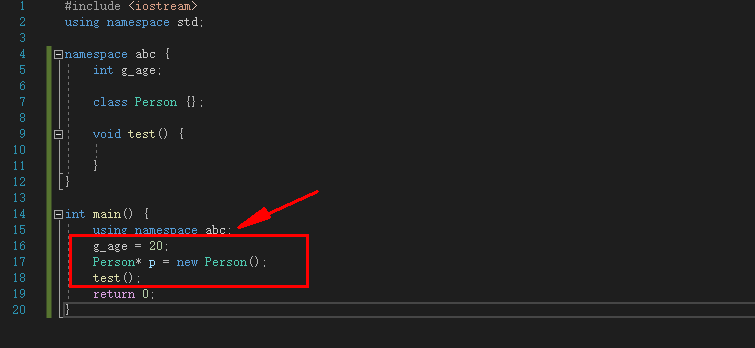``````#include <iostream>
using namespace std;

int g_age;

namespace abc {
int g_age;

class Person {};

void test() {

}
}

int main() {
::g_age = 20;
using namespace abc;
::abc::g_age = 70;
Person* p = new Person();
test();
return 0;
}``````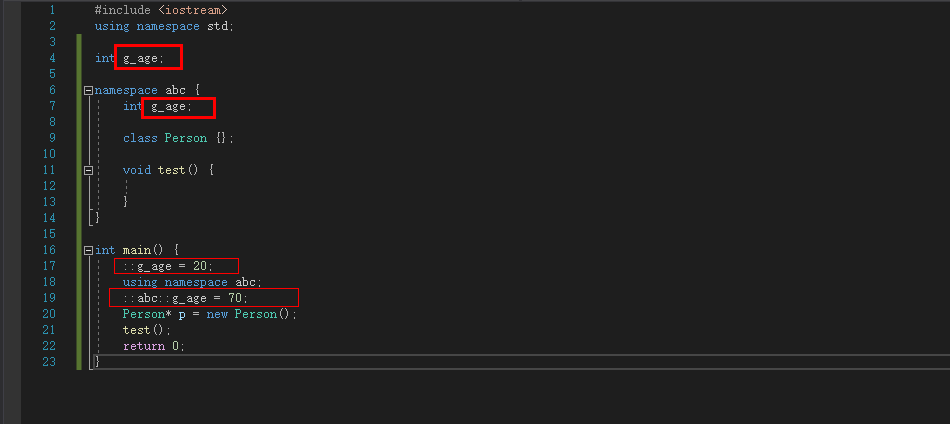## 继承

``````#include <iostream>
using namespace std;

class Person {
public:
int age;
void run() {
cout << "Person::run()" << endl;
}
};

class Student : public Person {
public:
int class_num;
void study() {
cout << "Student::study()" << endl;
}
};

int main() {
Student student;
student.age = 20;
student.class_num = 10;
student.run();
student.study();
}``````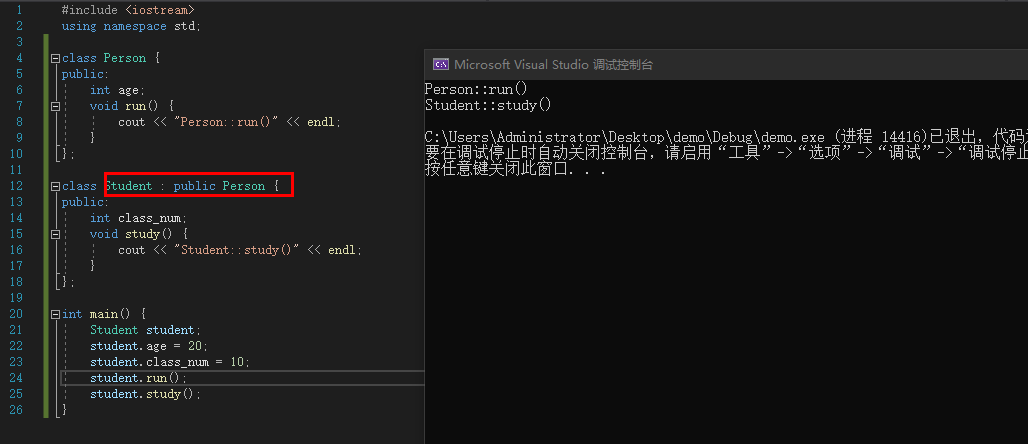• Student是子类（subclass，派生类）
• Person是父类（superclass，超类）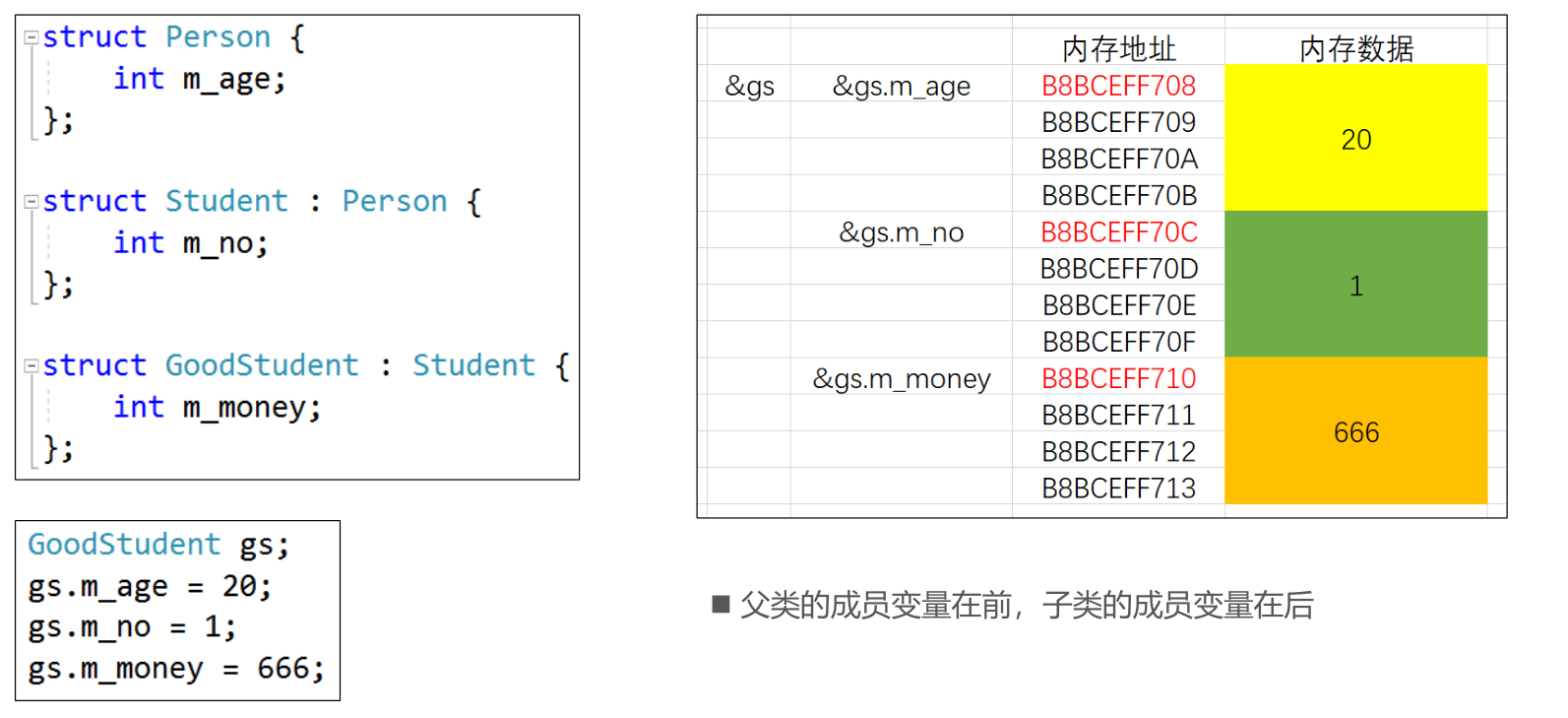## 成员访问权限

• public：公共的，任何地方都可以访问（struct默认）
• protected：子类内部、当前类内部可以访问
• private：私有的，只有当前类内部可以访问（class默认）

• 成员本身的访问权限
• 上一级父类的继承方式

## 初始化列表

• 一种便捷的初始化成员变量的方式
• 只能用在构造函数中
• 初始化顺序只跟成员变量的声明顺序有关

``````class Person {
public:
int m_age;
int m_height;
Person(int age, int height) : m_age(age), m_height(height) {

}
};``````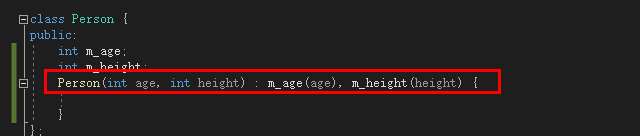``````class Person {
public:
int m_age;
int m_height;
Person(int age, int height) {
this->m_age = age;
this->m_height = height;
}
};``````

### 初始化列表与默认参数配合使用

``````#include <iostream>
using namespace std;

class Person {
public:
int m_age;
int m_height;
Person(int age = 0, int height = 0) : m_age(age), m_height(height) {
cout << m_age << " " << m_height << endl;
}
};

int main() {
Person person1;
Person person2(18);
Person person3(18, 20);
return 0;
}``````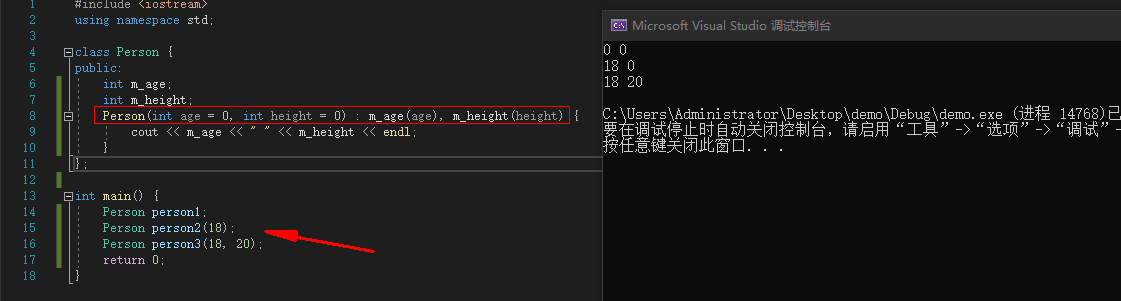• 初始化列表只能写在函数的实现中
• 默认参数只能写在函数的声明中

### 构造函数的互相调用

``````#include <iostream>
using namespace std;

class Person {
public:
int m_age;
int m_height;
Person() : Person(10,20) {

}
Person(int age , int height) : m_age(age), m_height(height) {
cout << m_age << " " << m_height << endl;
}
};

int main() {
Person person1;
return 0;
}``````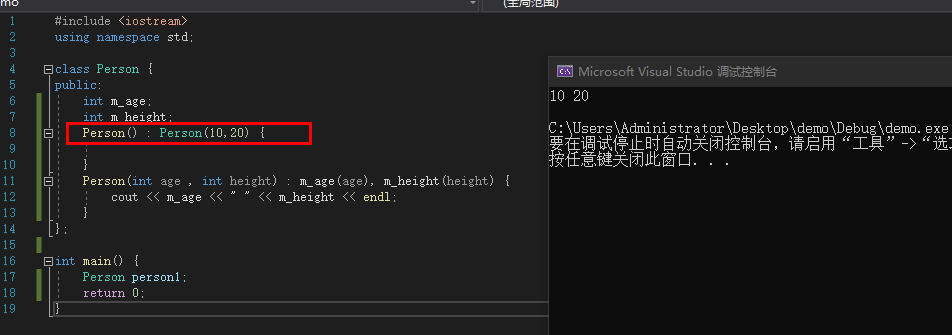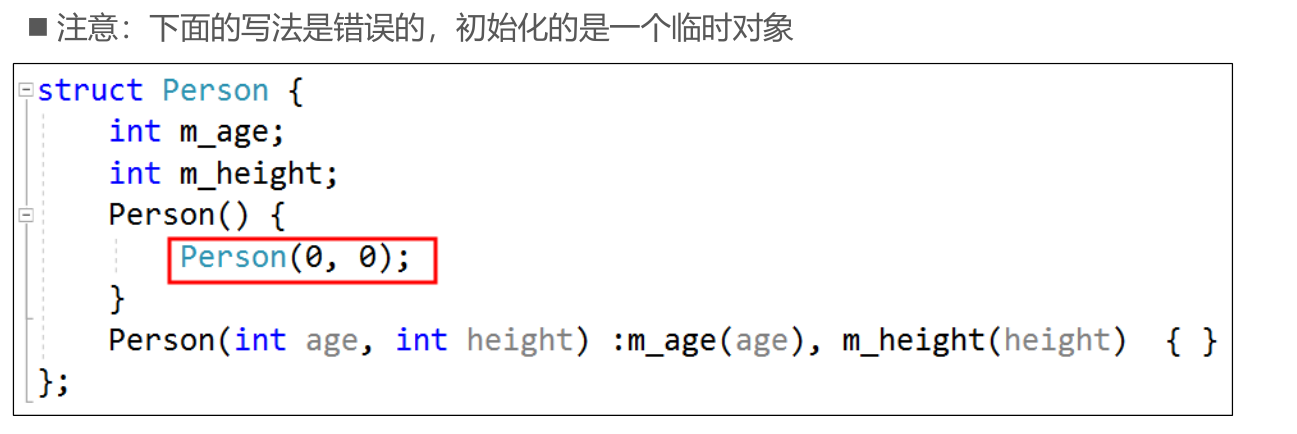### 父类的构造函数

• 子类的构造函数默认会调用父类的无参构造函数

• 如果子类的构造函数显式地调用了父类的有参构造函数，就不会再去默认调用父类的无参构造函数

• 如果父类缺少无参构造函数，子类的构造函数必须显式调用父类的有参构造函数

## 父类指针，子类指针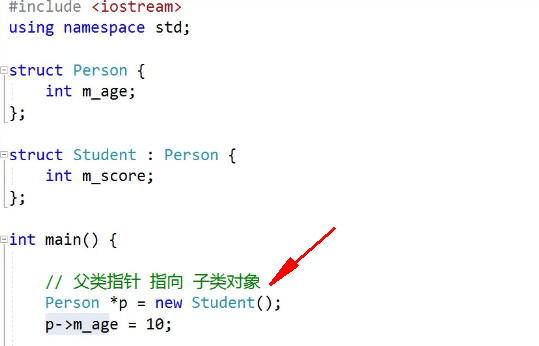## 多态

``````#include <iostream>
using namespace std;

class Dog {
public:
void speak() {
cout << "Dog::speak()" << endl;
}
void run() {
cout << "Dog::run()" << endl;
}
};

class Cat {
public:
void speak() {
cout << "Cat::speak()" << endl;
}
void run() {
cout << "Cat::run()" << endl;
}
};

class Pig {
public:
void speak() {
cout << "Pig::speak()" << endl;
}
void run() {
cout << "Pig::run()" << endl;
}
};

void liu(Dog* p) {
p->run();
p->speak();
}

void liu(Cat* p) {
p->run();
p->speak();
}

void liu(Pig* p) {
p->run();
p->speak();
}

int main() {
liu(new Dog());
liu(new Cat());
liu(new Pig());
return 0;
}``````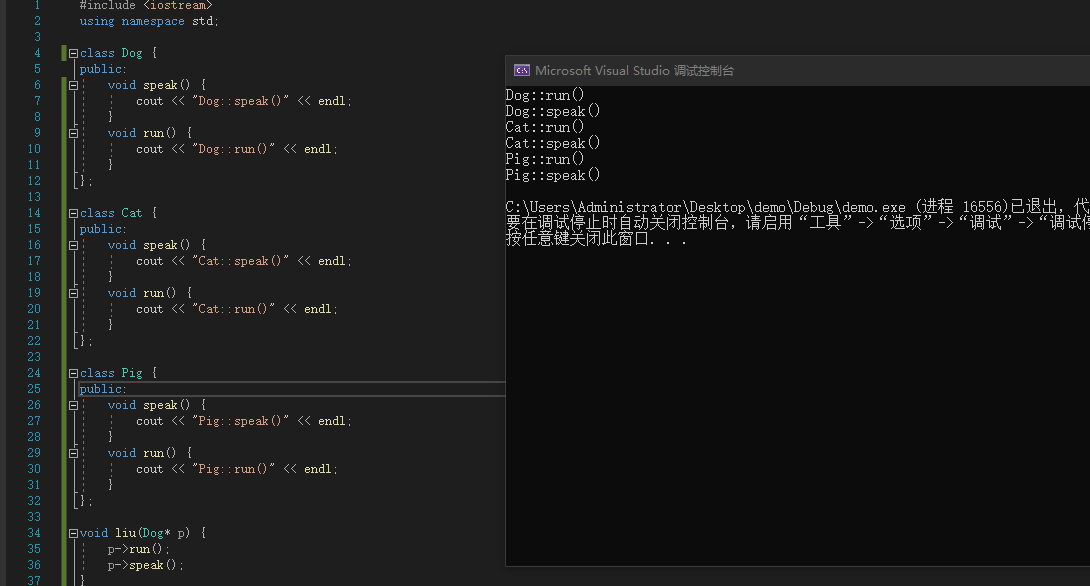``````#include <iostream>
using namespace std;

class Animal {
public:
void speak() {
cout << "Animal::speak()" << endl;
}
void run() {
cout << "Animal::run()" << endl;
}
};

class Dog : public Animal{
public:
void speak() {
cout << "Dog::speak()" << endl;
}
void run() {
cout << "Dog::run()" << endl;
}
};

class Cat : public  Animal {
public:
void speak() {
cout << "Cat::speak()" << endl;
}
void run() {
cout << "Cat::run()" << endl;
}
};

class Pig : public  Animal {
public:
void speak() {
cout << "Pig::speak()" << endl;
}
void run() {
cout << "Pig::run()" << endl;
}
};

//父指针指向子类对象
void liu(Animal * p) {
p->run();
p->speak();
}

int main() {
liu(new Dog());
liu(new Cat());
liu(new Pig());
return 0;
}``````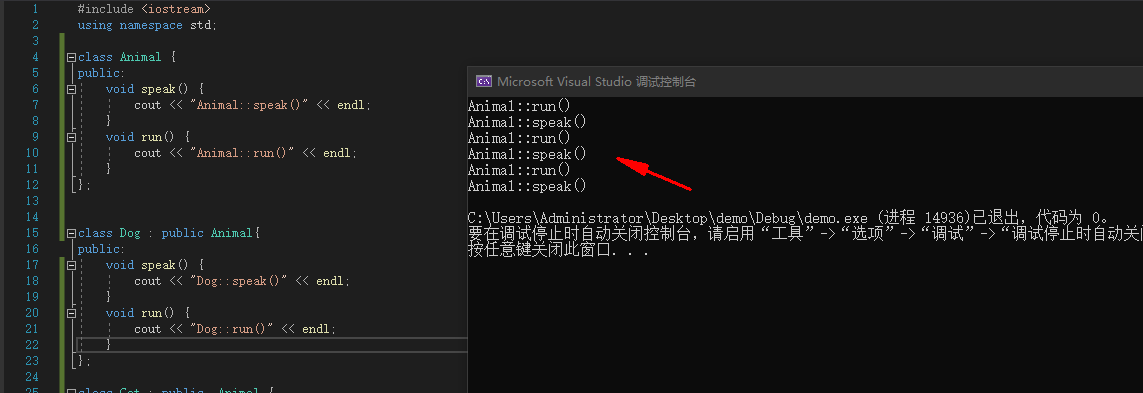• 子类重写父类的成员函数（override）
• 父类指针指向子类对象
• 利用父类指针调用重写的成员函数

• 同一操作作用于不同的对象，可以有不同的解释，产生不同的执行结果
• 在运行时，可以识别出真正的对象类型，调用对应子类中的函数

### 虚函数

C++中的多态通过虚函数（virtual function）来实现。

``````#include <iostream>
using namespace std;

class Animal {
public:
virtual void speak() {
cout << "Animal::speak()" << endl;
}
virtual void run() {
cout << "Animal::run()" << endl;
}
};

class Dog : public Animal{
public:
void speak() {
cout << "Dog::speak()" << endl;
}
void run() {
cout << "Dog::run()" << endl;
}
};

class Cat : public  Animal {
public:
void speak() {
cout << "Cat::speak()" << endl;
}
void run() {
cout << "Cat::run()" << endl;
}
};

class Pig : public  Animal {
public:
void speak() {
cout << "Pig::speak()" << endl;
}
void run() {
cout << "Pig::run()" << endl;
}
};

void liu(Animal * p) {
p->run();
p->speak();
}

int main() {
liu(new Dog());
liu(new Cat());
liu(new Pig());
return 0;
}``````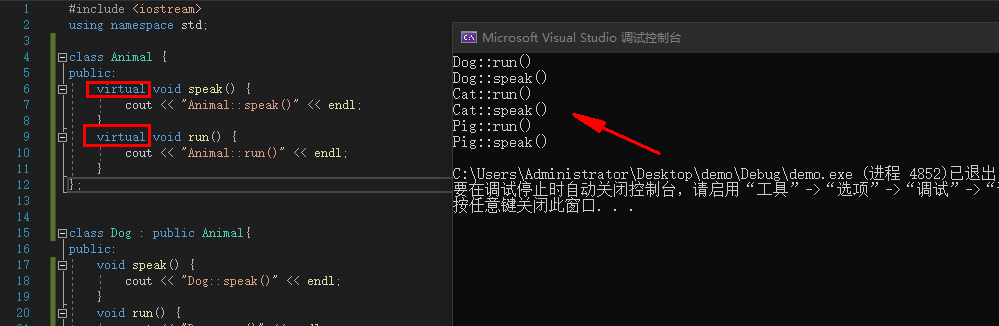### 虚表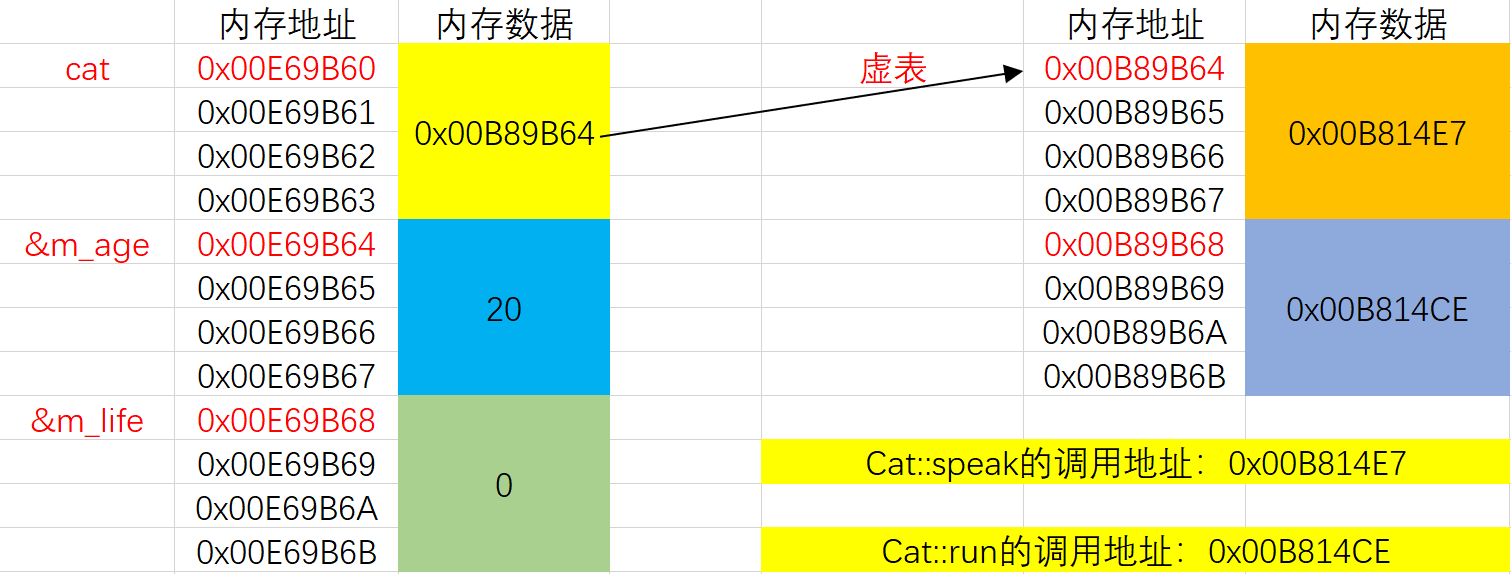### 虚析构函数

delete父类指针时，才会调用子类的析构函数，保证析构的完整性。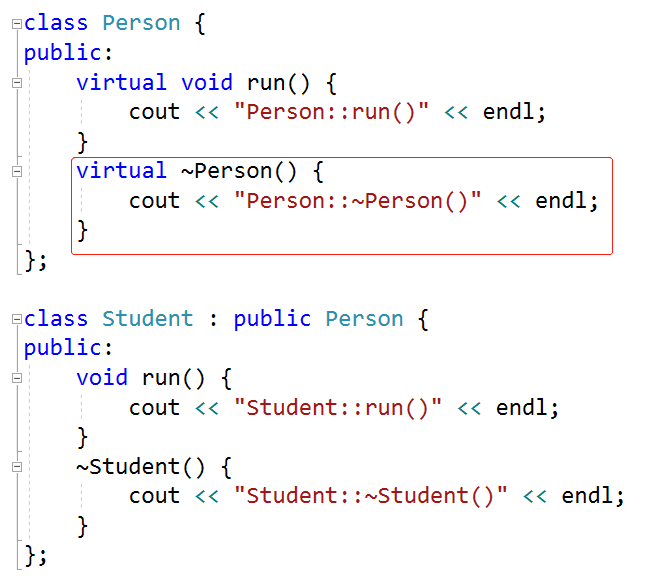### 纯虚函数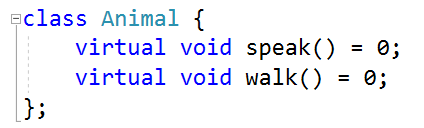• 含有纯虚函数的类，不可以实例化（不可以创建对象）
• 抽象类也可以包含非纯虚函数、成员变量
• 如果父类是抽象类，子类没有完全重写纯虚函数，那么这个子类依然是抽象类

## 静态成员

### 静态成员变量

• 存储在数据段（全局区，类似于全局变量），整个程序运行过程中只有一份内存
• 对比全局变量，它可以设定访问权限（public、protected、private），达到局部共享的目的
• 必须初始化，必须在类外面初始化，初始化时不能带static，如果类的声明和实现分离（在实现.cpp中初始化）

``````#include <iostream>
using namespace std;

class Car {
public:
static int m_price;
void run() {
cout << "run()" << endl;
}
};

//在类外面初始化静态成员变量
int Car::m_price = 10;

int main() {
Car car1;
car1.m_price = 100;
cout << car1.m_price << endl;
cout << Car::m_price << endl;
return 0;
}``````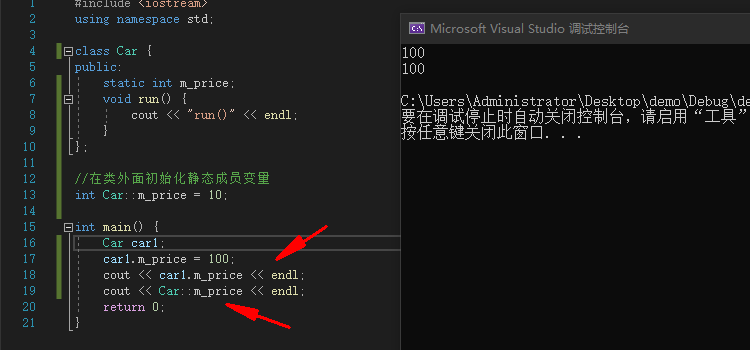### 静态成员函数

• 内部不能使用this指针（this指针只能用在非静态成员函数内部）
• 不能是虚函数（虚函数只能是非静态成员函数）
• 内部不能访问非静态成员变量\函数，只能访问静态成员变量\函数
• 非静态成员函数内部可以访问静态成员变量\函数
• 构造函数、析构函数不能是静态
• 当声明和实现分离时，实现部分不能带static

``````#include <iostream>
using namespace std;

class Car {
public:
static int m_price;
static void run() {
cout << "run()" << endl;
}
};

//在类外面初始化静态成员变量
int Car::m_price = 10;

int main() {
Car car1;
car1.run();
Car * p = new Car();
p->run();
Car::run();
return 0;
}``````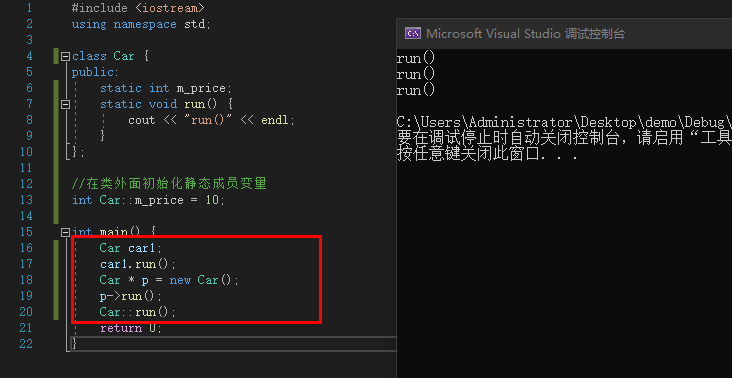### 静态成员应用

``````#include <iostream>
using namespace std;

class Car {
private:
static int m_count;
public:
Car() {
m_count++;
}
~Car() {
m_count--;
}
static int getCount() {
return m_count;
}
};

//在类外面初始化静态成员变量
int Car::m_count = 0;

Car g_car;  //创建了第一个Car对象

int main() {
Car car1;  //创建了第二个Car对象
Car * p = new Car();  //创建了第三个Car对象
cout << Car::getCount() << endl;
return 0;
}``````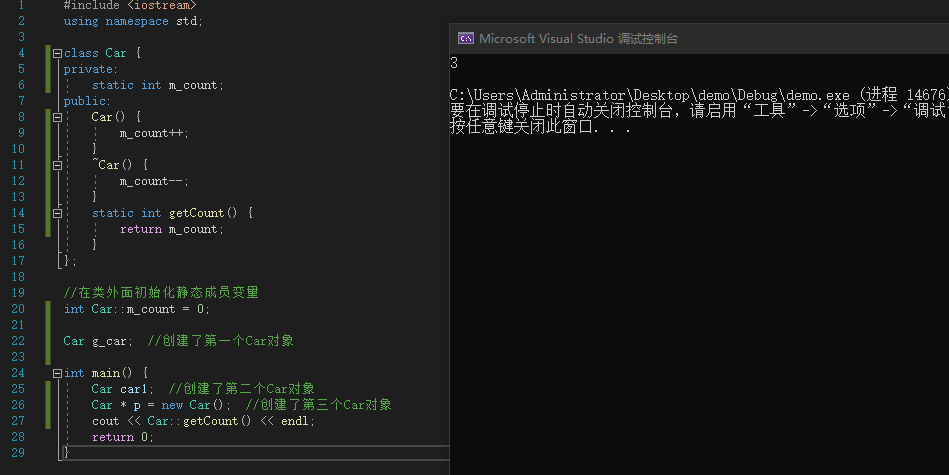## const 成员

const成员：被const修饰的成员变量、非静态成员函数。

const成员变量

• 必须初始化（类内部初始化），可以在声明的时候直接初始化赋值

• 非static的const成员变量还可以在初始化列表中初始化

const成员函数（非静态）

const关键字写在参数列表后面，函数的声明和实现都必须带const

• 内部不能修改非static成员变量
• 内部只能调用const成员函数、static成员函数
• 非const成员函数可以调用const成员函数

const成员函数和非const成员函数构成重载

const对象（指针）只能调用const成员函数、static成员函数

## 引用类型成员

• 在声明的时候直接初始化
• 通过初始化列表初始化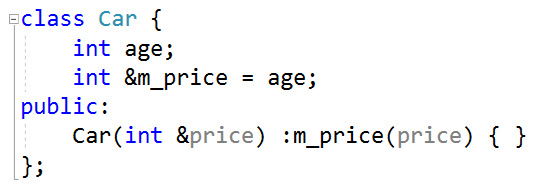## 拷贝构造函数（Copy Constructor）

``````#include <iostream>
using namespace std;

class Car {
int m_price;
int m_length;
public:
Car(int price = 0, int length = 0) :m_price(price), m_length(length) {
cout << "Car(int price = 0, int length = 0)" << endl;
}

//拷贝构造函数，格式固定
Car(const Car &car) {
cout << "Car(const Car &car)" << endl;
}

void display() {
cout << "price=" << m_price << ", length=" << m_length << endl;
}
};

int main() {
Car car1;
Car car2(100);
Car car3(100, 50);

//利用已经存在的car3对象创建了一个car4新对象
//car4初始化时只会调用拷贝构造函数
Car car4(car3);
return 0;
}``````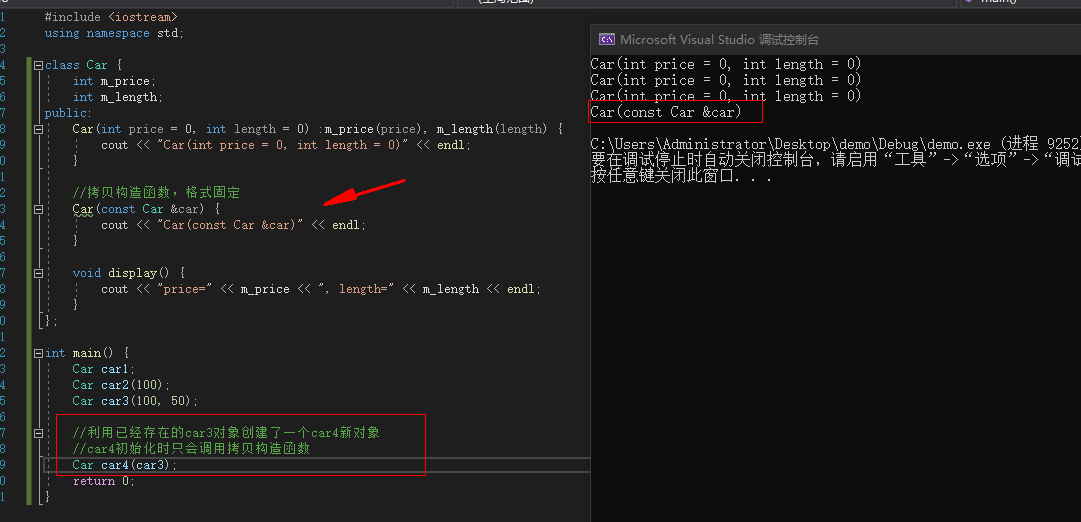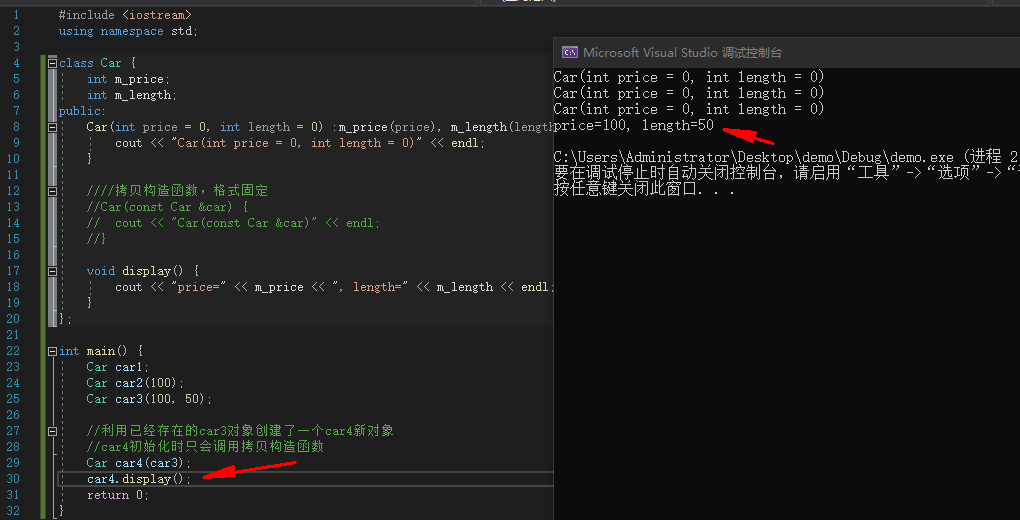### 调用父类的拷贝构造函数

``````#include <iostream>
using namespace std;

class Person {

public:
int m_age;
Person(int age=0) :m_age(age){}
//父类的拷贝构造函数
Person(const Person &person) :m_age(person.m_age){}
};

class Student : public Person {

public:
int m_score;
//子类调用父类的构造函数
Student(int age=0, int score=0) :Person(age), m_score(score){}
//子类调用父类的拷贝构造函数
Student(const Student &student) :Person(student), m_score(student.m_score){}
};

int main(){
Student student1(10, 100);
Student student2(student1);
cout << student2.m_age << endl;
cout << student2.m_score << endl;
}``````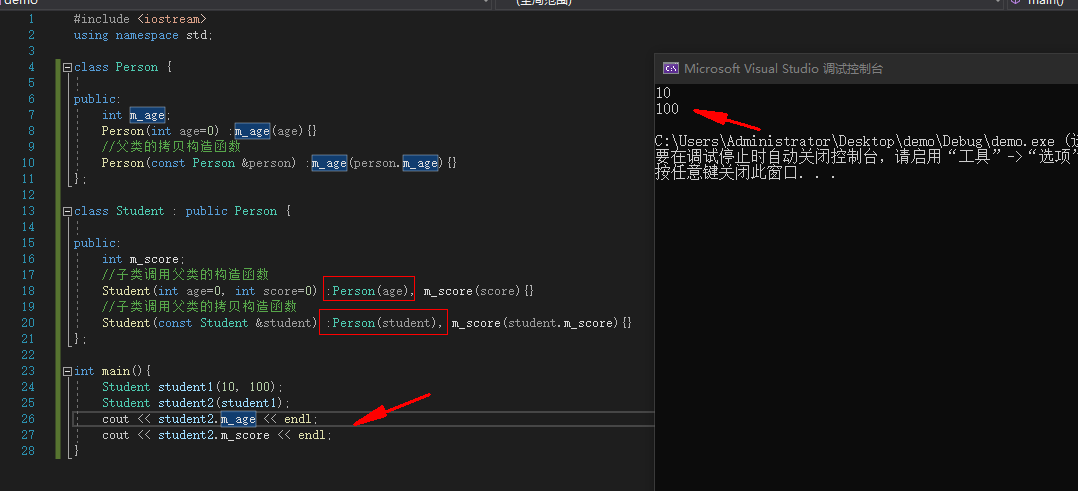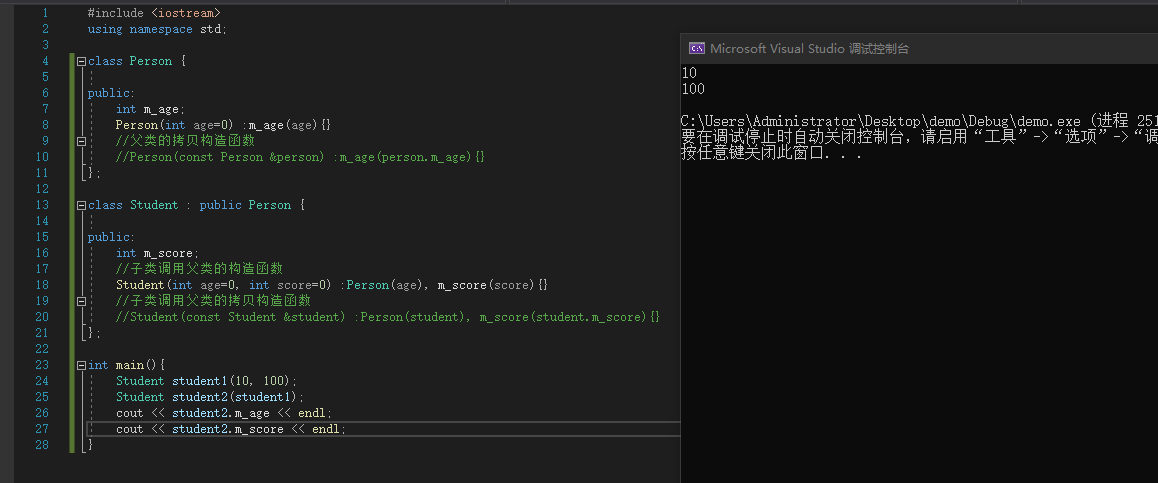### 浅拷贝和深拷贝

• 将一个对象中所有成员变量的值拷贝到另一个对象
• 如果某个成员变量是个指针，只会拷贝指针中存储的地址值，并不会拷贝指针指向的内存空间
• 可能会导致堆空间多次free的问题

• 将指针类型的成员变量所指向的内存空间，拷贝到新的内存空间

## 友元

• 如果将函数A（非成员函数）声明为类C的友元函数，那么函数A就能直接访问类C对象的所有成员
• 如果将类A声明为类C的友元类，那么类A的所有成员函数都能直接访问类C对象的所有成员
• 友元破坏了面向对象的封装性，但在某些频繁访问成员变量的地方可以提高性能

``````#include <iostream>
using namespace std;

class Point {
int m_x;
int m_y;
public:
int getX() {
return m_x;
};
int getY() {
return m_y;
};
Point(int x , int y): m_x(x),m_y(y){}
void display() {
cout << "(" << m_x << ", " << m_y << ")" <<endl;
}
};

Point add(Point p1, Point p2) {
return Point(p1.getX() + p2.getX(), p1.getY() + p2.getY());
}

int main() {
Point P1(10, 20);
Point P2(20, 30);
P3.display();
return 0;
}``````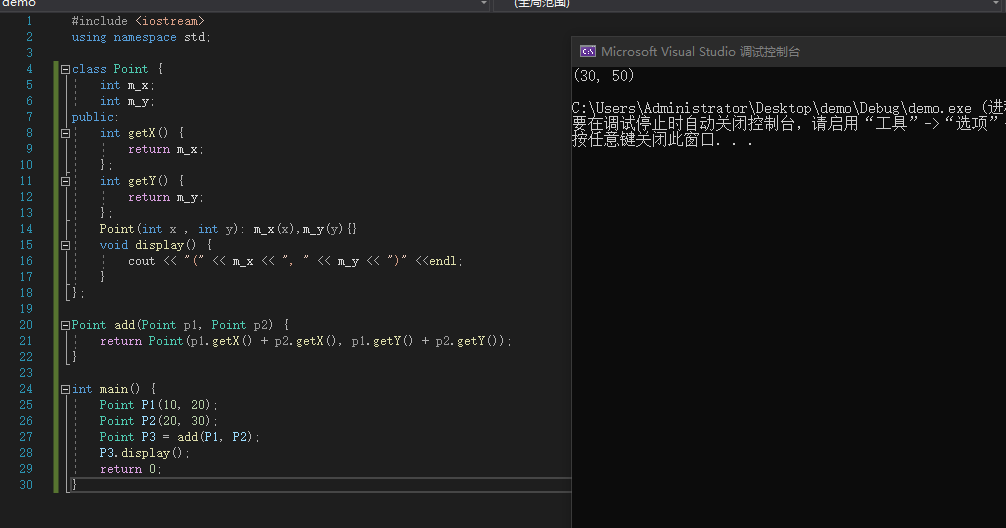``````#include <iostream>
using namespace std;

class Point {
friend Point add(Point p1, Point p2);
int m_x;
int m_y;
public:
int getX() {
return m_x;
};
int getY() {
return m_y;
};
Point(int x , int y): m_x(x),m_y(y){}
void display() {
cout << "(" << m_x << ", " << m_y << ")" <<endl;
}
};

Point add(Point p1, Point p2) {
return Point(p1.m_x + p2.m_x, p1.m_y + p2.m_y);
}

int main() {
Point P1(10, 20);
Point P2(20, 30);
P3.display();
return 0;
}``````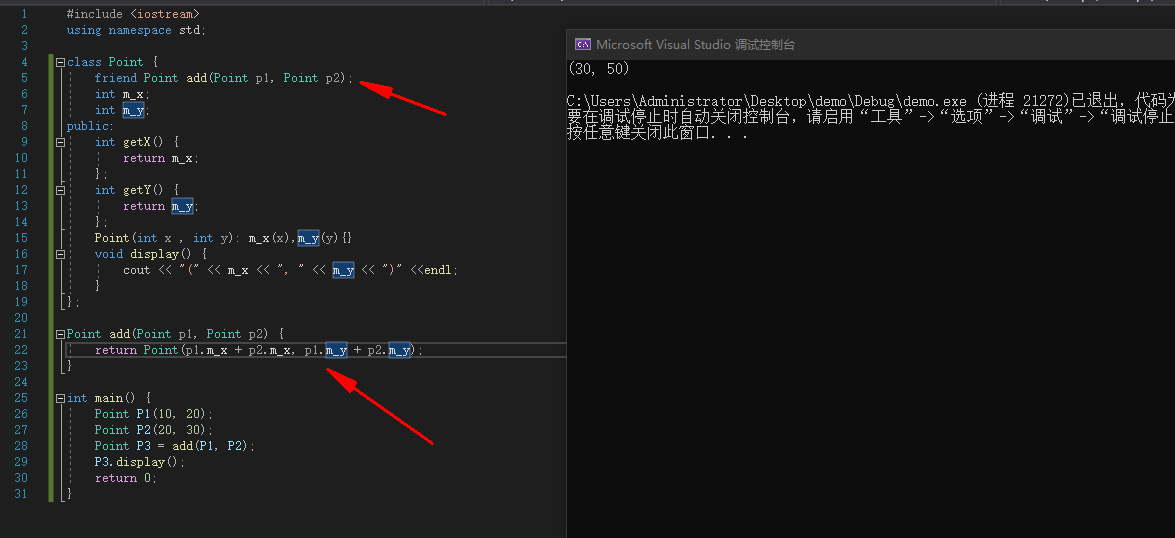## 内部类

• 支持public、protected、private权限

• 成员函数可以直接访问其外部类对象的所有成员（反过来则不行）

• 成员函数可以直接不带类名、对象名访问其外部类的static成员

• 不会影响外部类的内存布局

• 可以在外部类内部声明，在外部类外面进行定义

## 局部类

• 作用域仅限于所在的函数内部
• 其所有的成员必须定义在类内部，不允许定义static成员变量
• 成员函数不能直接访问函数的局部变量（static变量除外）

# 其他语法

## 运算符重载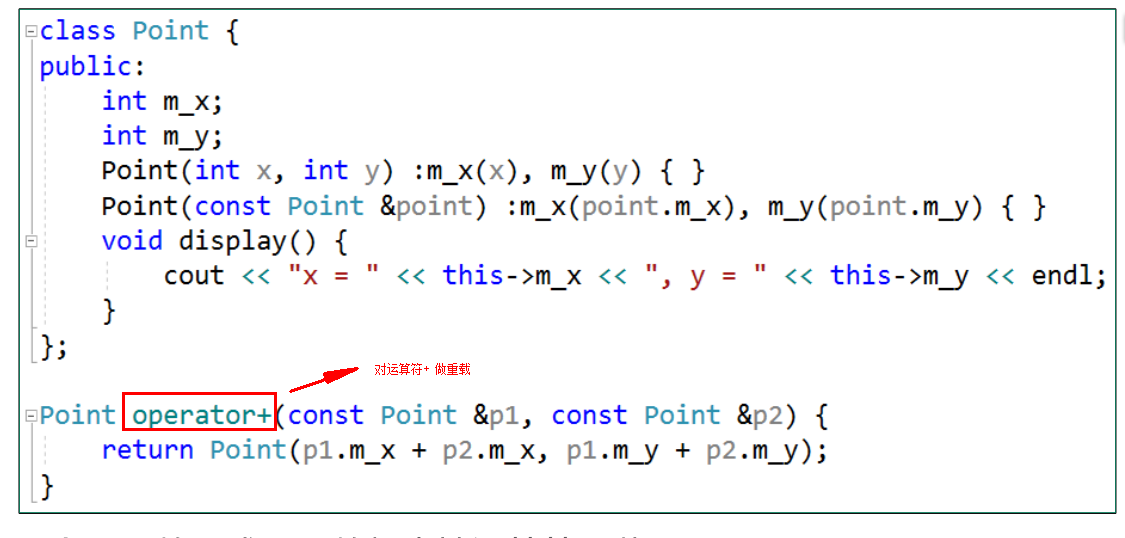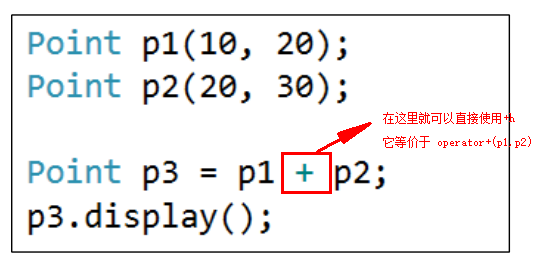## 异常

• 异常是一种在程序运行过程中可能会发生的错误（比如内存不够）
• 异常没有被处理，会导致程序终止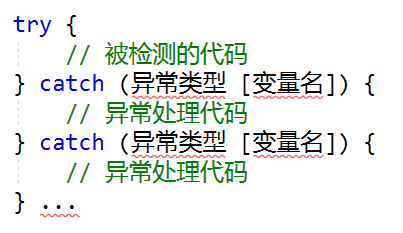throw异常后，会在当前函数中查找匹配的catch，找不到就终止当前函数代码，去上一层函数中查找。如果最终都找不到匹配的catch，整个程序就会终止。

``````#include <iostream>
using namespace std;

int divide(int v1, int v2) {
if (v2 == 0)
{
throw "不能除以0";
}
return v1 / v2;
}

int main() {
try {
int a = 10;
int b = 0;
divide(a, b);
}
catch (...) {
cout << "运算错误" << endl;
}

return 0;
}``````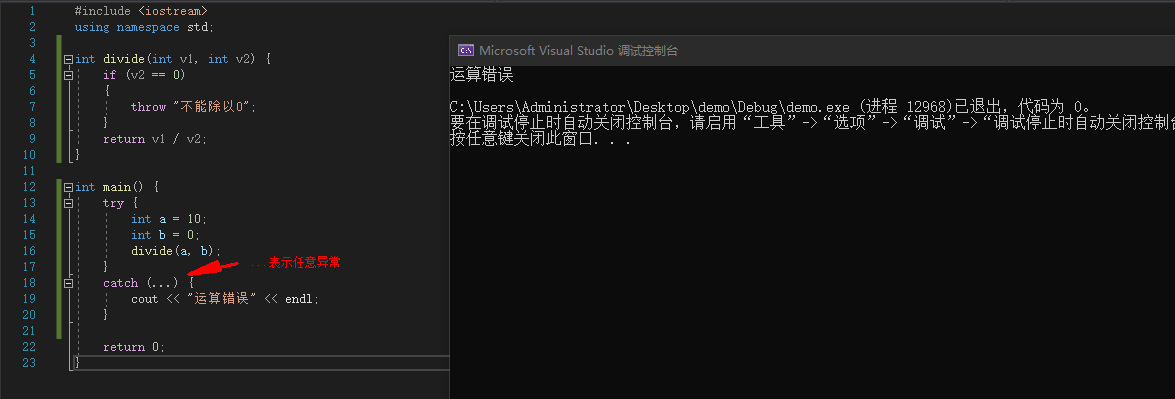``````#include <iostream>
using namespace std;

int divide(int v1, int v2) {
if (v2 == 0)
{
throw "不能除以0";
}
return v1 / v2;
}

int main() {
try {
int a = 10;
int b = 0;
divide(a, b);
}
catch (const char * exception) {
cout << "运算错误:" << exception << endl;
}

return 0;
}``````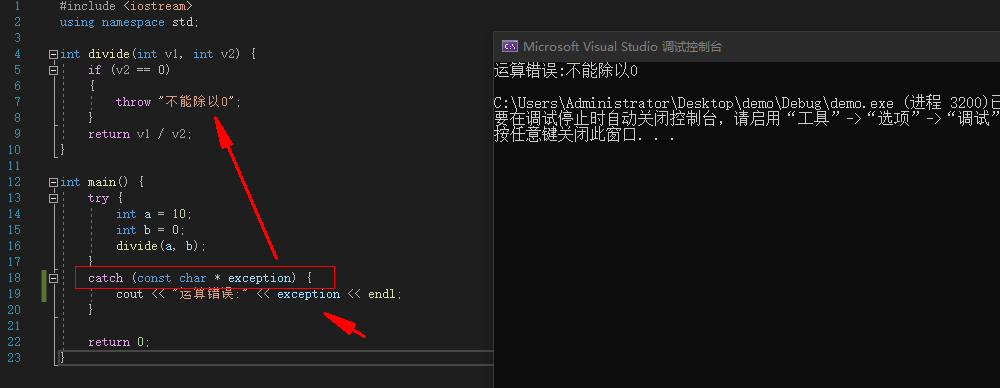``````#include <iostream>
using namespace std;

class Exception {
public:
virtual const char * what() = 0;
};

class DivideException : public Exception {
public:
const char* what() {
return "不能除以0";
}
};

class AddException : public Exception {
public:
const char* what() {
return "加法有问题";
}
};

int divide(int v1, int v2) {
if (v2 == 0)
{
throw DivideException();
}
return v1 / v2;
}

int main() {
try {
int a = 10;
int b = 0;
divide(a, b);
}
catch (DivideException exception) {
cout << "产生了异常:" << exception.what() << endl;
}

return 0;
}``````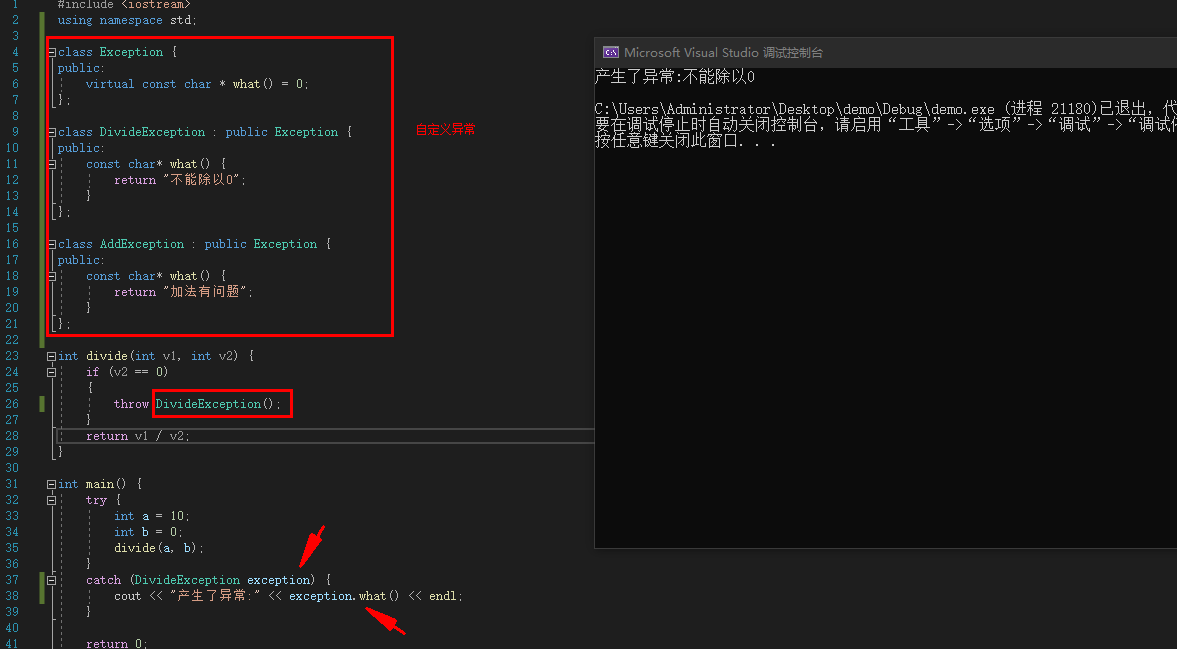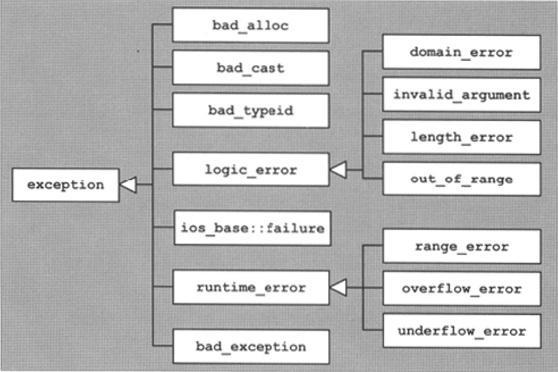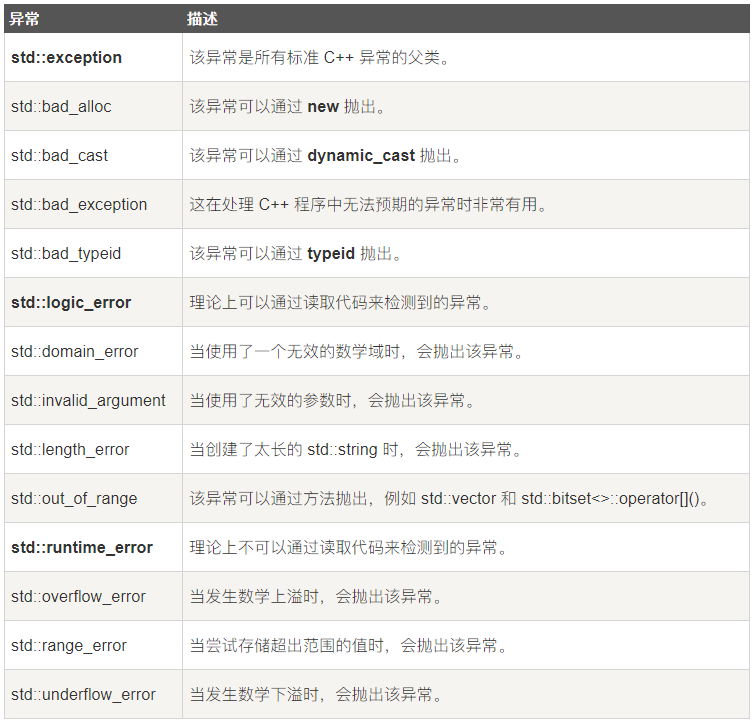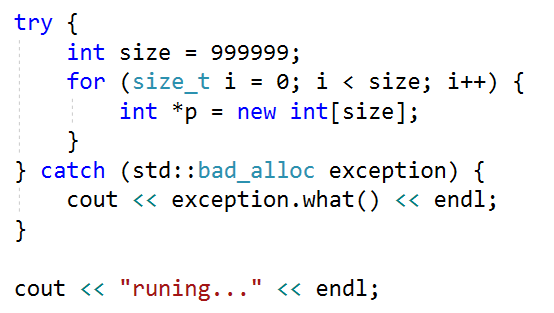目录